## Python库的选择

1. csv文件的读取；
2. 对读取的数据，按照我们要分析的指标进行数据处理和指标计算；
3. 根据数据分析的结果，生成可视化的数据图表；
4. 通过web页面展示数据分析结果报告；

### 1.数据处理和分析库

Pandas是基于Numpy数组构建的，但二者最大的不同是pandas是专门为处理表格和混杂数据设计的，比较契合统计分析中的表结构，而numpy更适合处理统一的数值数组数据。

### 2.数据可视化库

• Matplotlib
• Seanborn
• Pyecharts

Matplotlib

Matplotlib可以说是Python数据可视化库的鼻祖了，他是Python编程语言及其数值计算包NumPy的可视化操作界面，其中pyplot是matplotlib的一个模块，提供了类似MATLAB的接口。其可以和Numpy、Pandas无缝结合，但一些图标的样式不够美观，而且原生不支持生成动态可交互的图表，虽然可以通过改变使用的后端来实现，但相对还是比较麻烦一些，而且如果想要在一个web页面中实现一个动态可交互的图表，目前没有什么特别好的办法，最近matplotlib在更面向web交互方面有了很多进展，比如新的HTML5/Canvas后端，可以从如下地址了解一下：

```http://code.google.com/p/mplh5canvas/
```

Seanborn

Seaborn跟matplotlib最大的区别就是它的默认绘图风格和色彩搭配都具有现代美感，其实他是在matplotlib的基础上进行了更高级的API封装，让你能用更少的代码去调用matplotlib的方法，从而使得作图更加容易。但matplotlib存在的动态交互性的问题他同样存在。

Pyecharts

• Django

## 开始我们的数据可视化分析之旅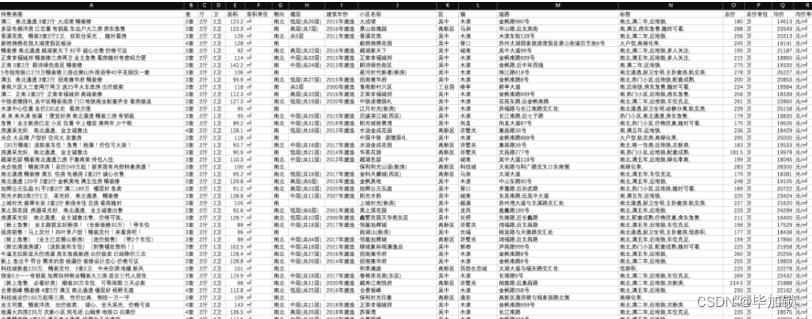• Bar（柱状图）

• 数据计算处理
• 数据可视化处理

1. 根据原始数据表中的”小区名称“字段进行group by；
2. 对每个分组，对”均价“字段求平均值；
3. 对上述结果的”均价“字段按降序进行排序；
4. 对排序结果取前10项结果；

def unit_price_analysis_by_estate(df,isembed):
#获取要分析的数据列
analysis_df = df.loc[:,[‘小区名称’,’均价’]]
analysis_df.loc[:,’小区名称’] = analysis_df.loc[:,’小区名称’].astype(‘str’)
#对小区名称分组，然后按照分组计算单价均价
group = analysis_df.groupby(‘小区名称’,as_index=False)
group_df = group.mean()
group_df.loc[:,’均价’] = group_df.loc[:,’均价’].astype(‘int’)
#按照均价列降序排序
group_df.sort_values(‘均价’,ascending=False, inplace=True)
#取Top10
#为了横向柱状图展示，再从低到高排序一下
top10_df.sort_values(‘均价’,ascending=True,inplace=True)
……

bar = (
Bar(init_opts=opts.InitOpts(width=”1500px”))
.reversal_axis()
.set_series_opts(label_opts=opts.LabelOpts(position=”right”))
.set_global_opts(title_opts=opts.TitleOpts(title=”苏州各小区二手房房价TOP10″),
xaxis_opts=opts.AxisOpts(axislabel_opts={‘interval’:’0′}),
legend_opts=opts.LegendOpts(is_show=False))
)

top10_color_function = “””
function (params) {
if (params.value > 58000 && params.value < 59000) {
return ‘red’;
} else if (params.value > 59000 && params.value < 60000) {
return ‘blue’;
}else if (params.value > 60000 && params.value < 61000){
return ‘green’
}else if (params.value > 61000 && params.value < 61800){
return ‘purple’
}else if (params.value > 61800 && params.value < 70000){
return ‘brown’
}else if (params.value > 70000 && params.value < 73000){
return ‘gray’
}else if (params.value > 73000 && params.value < 79000){
return ‘orange’
}else if (params.value > 79000 && params.value < 85000){
return ‘pink’
}else if (params.value > 85000 && params.value < 100000){
return ‘navy’
}
return ‘gold’;
}
“””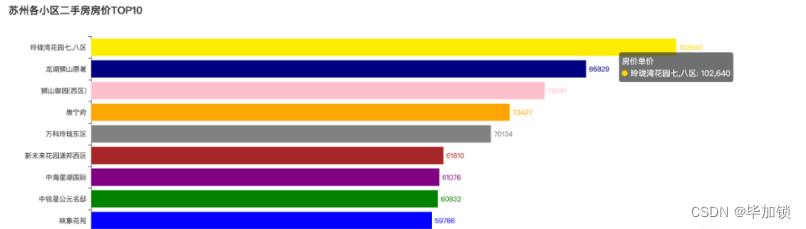• Pie（饼图）

1. 因为原数据表中没有待售房屋数这一列，因此我们先增加一列，用于后续计算；
2. 对建筑年份列进行group by；
3. 对每个分组进行统计计数，结果写入新增加的待售房屋数列；

return 0
def sale_estate_analysis_by_year(df,isembed):
#增加一列待售房屋数，初始值均为0
#获取要用作数据分析的两列：建筑年份和待售房屋数
analysis_df = df.loc[:,[‘建筑年份’,’待售房屋数’]]
#因为建筑年份列有空值，先预处理一下
analysis_df.dropna(inplace=True)
#按照建筑年份进行分组
group = analysis_df.groupby(‘建筑年份’,as_index=False)
#对每个分组进行统计计数
group_df = group.count()
group_df.loc[:,’待售房屋数’] = group_df.loc[:,’待售房屋数’].astype(‘int’)
……

pie = Pie(init_opts=opts.InitOpts(width=’800px’, height=’600px’, bg_color=’white’))
,center=[‘50%’, ‘50%’]
,label_opts=opts.LabelOpts(
position=”outside”,
formatter=”{b}:{c}:{d}%”,)
).set_global_opts(
title_opts=opts.TitleOpts(title=’苏州二手房不同建筑年份的待售数量’, pos_left=’300′, pos_top=’20’,
title_textstyle_opts=opts.TextStyleOpts(color=’black’, font_size=16)),
legend_opts=opts.LegendOpts(is_show=False))

```    [list(z) for z in zip(group_df['建筑年份'].tolist()
```

• Histogram（直方图）

1. 将要分析的数据字段进行分段；
2. 对每个分段，计算该分段里的分布数量；

import numpy as np
def unit_price_analysis_by_histogram(df,isembed):
hist,bin_edges = np.histogram(df[‘均价’],bins=100)
bar = (
Bar()
.set_global_opts(
title_opts=opts.TitleOpts(title=’苏州二手房房价-单价分布-直方图’,pos_left=’center’),
legend_opts=opts.LegendOpts(is_show=False)
)
)
……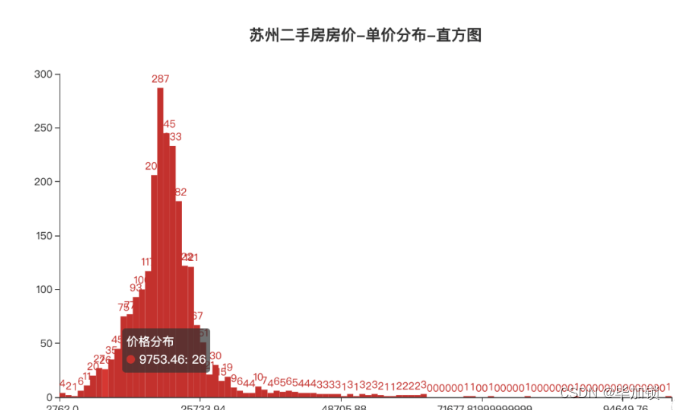• Scatter（散点图）

df.sort_values(‘面积’,ascending=True, inplace=True)
square = df[‘面积’].to_list()
unit_price = df[‘均价’].to_list()
scatter = (
Scatter()
series_name=”,
y_axis=unit_price,
symbol_size=4,
label_opts=opts.LabelOpts(is_show=False)
)
.set_global_opts(
xaxis_opts=opts.AxisOpts(type_=’value’),
yaxis_opts=opts.AxisOpts(type_=’value’),
title_opts=opts.TitleOpts(title=’苏州二手房面积-单价关系图’,pos_left=’center’)
)
)

• Map（地图）

1. 获取数据源中的区和均价两个字段；
2. 对区字段进行group by；
3. 对分组后的数据求平均值；
4. 为适配地图组件的行政区划名称，对区字段进行一下转换处理；
5. 将数据转换成地图组件需要的二维数组的格式；

def transform_name(row):
district_name = row[‘区’].strip()
if district_name == ‘吴中’ or district_name == ‘相城’ or district_name == ‘吴江’ or district_name == ‘虎丘’ or district_name == ‘姑苏’ or district_name == ‘工业园’:
district_name = district_name + ‘区’
if district_name == ‘常熟’ or district_name == ‘张家港’ or district_name == ‘太仓’:
district_name = district_name + ‘市’
return district_name
data = []
#获取要分析的数据列
analysis_df = df.loc[:,[‘区’,’均价’]]
#按区列分组
group_df = analysis_df.groupby(‘区’,as_index=False)
#根据分组对均价列求平均值
group_df = group_df.mean(‘均价’)
#print(group_df)
#将区的名字做一下转换，为下面的地图匹配做准备
group_df[‘区’] = group_df.apply(transform_name,axis=1)
group_df.loc[:,’均价’] = group_df.loc[:,’均价’].astype(‘int’)
#将数据转换成map需要的数据格式
for index,row in group_df.iterrows():
district_array = [row[‘区’],row[‘均价’]]
data.append(district_array)

map = (
Map()
.set_global_opts(
title_opts=opts.TitleOpts(title=’苏州各区域二手房房价地图’,pos_left=’center’),
visualmap_opts=opts.VisualMapOpts(max_=26000),
legend_opts=opts.LegendOpts(is_show=False)
)
)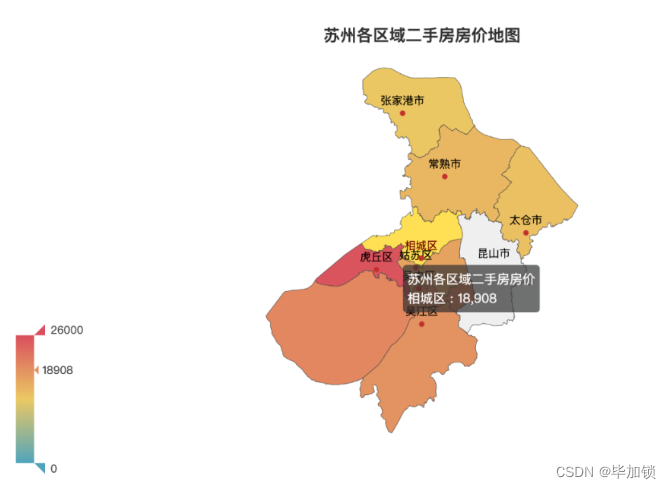• WordCloud（词云图）

txt = ”
for index,row in df.iterrows():
txt = txt+ str(row[‘待售房屋’]) + ‘;’+ str(row[‘标签’]) + ‘\n’
word_weights = jieba.analyse.extract_tags(txt,topK=100,withWeight=True)
word_cloud=(
WordCloud()
.set_global_opts(
title_opts=opts.TitleOpts(
title=’苏州二手房销售热度词’,
title_textstyle_opts=opts.TextStyleOpts(font_size=23),
pos_left=’center’
)
)
)

## 生成动态可交互的Web数据分析报告

1. 启动一个web服务；
2. 读取我们要分析的原始数据；
3. 实现一个函数负责将读取的数据传给不同的数据图表生成函数，拿到生成的数据图表对象，然后调用模版进行渲染；
4. 绑定一个url路由关系，映射到步骤三的函数；
• 用来渲染生成最终数据分析报告的HTML文件，这个文件主要干如下几件事：
1. 对每个数据图表定义一个div；
2. 使用ECharts组件对div进行初始化；
• HTML渲染和计算所依赖的静态资源文件，主要有如下三个：
1. echarts-wordcloud.min.js，主要用于词云图生成;
2. jiang1_su1_su1_zhou1.js，主要用于苏州地图生成；
3. echarts.min.js，是所有数据图表依赖的基础js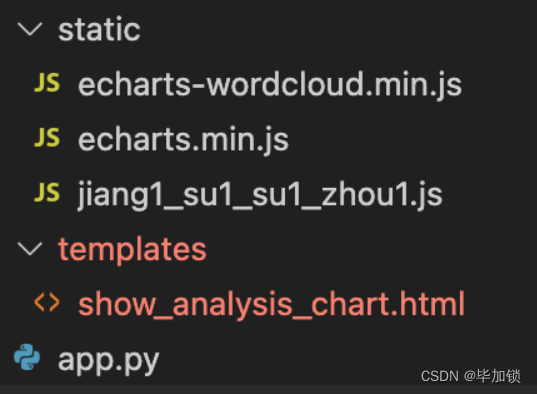import drawChart as dbc
import pandas as pd
#读取要分析的数据
fpath = ‘path/filename.xlsx’
#绑定url映射关系
@app.route(“/show_all_analysis_chart”)
def show_all_analysis_chart():
#获取按面积区间的单价分析数据
unit_price_analysis_by_square = dbc.unit_price_analysis_by_square(df,False)
#获取按室区分的单价分析数据
unit_price_analysis_by_layout = dbc.unit_price_analysis_by_layout(df,False)
#获取苏州各小区二手房房价TOP10
unit_price_analysis_by_estate = dbc.unit_price_analysis_by_estate(df,False)
#获取不同建筑年份的待售房屋数
sale_estate_analysis_by_year = dbc.sale_estate_analysis_by_year(df,False)
#苏州二手房房价-单价分布-直方图
unit_price_analysis_by_histogram = dbc.unit_price_analysis_by_histogram(df,False)
#苏州二手房房价-总价分布-直方图
total_price_analysis_by_histogram = dbc.total_price_analysis_by_histogram(df,False)
#苏州二手房面积-单价关系图
unit_price_analysis_by_scatter = dbc.unit_price_analysis_by_scatter(df,False)
#苏州二手房销售热度词
hot_word_analysis_by_wordcloud = dbc.hot_word_analysis_by_wordcloud(df,False)
#苏州各区域二手房房价
unit_price_analysis_by_map = dbc.unit_price_analysis_by_map(df,False)
return render_template(“show_analysis_chart.html”,
unit_price_analysis_by_square_option = unit_price_analysis_by_square.dump_options(),
unit_price_analysis_by_layout_option = unit_price_analysis_by_layout.dump_options(),
unit_price_analysis_by_estate_option = unit_price_analysis_by_estate.dump_options(),
sale_estate_analysis_by_year_option = sale_estate_analysis_by_year.dump_options(),
unit_price_analysis_by_histogram_option = unit_price_analysis_by_histogram.dump_options(),
total_price_analysis_by_histogram_option = total_price_analysis_by_histogram.dump_options(),
unit_price_analysis_by_scatter_option = unit_price_analysis_by_scatter.dump_options(),
hot_word_analysis_by_wordcloud_option = hot_word_analysis_by_wordcloud.dump_options(),
unit_price_analysis_by_map_option = unit_price_analysis_by_map.dump_options()
)
#启动web应用
if __name__ == “__main__”:
app.run()

Html的核心代码如下：

<meta charset=”UTF-8″>
<title>苏州二手房数据分析报告</title>
<script type=”text/javascript” src=”/static/echarts.min.js”></script>
<script type=”text/javascript” src=”/static/echarts-wordcloud.min.js”></script>
<script type=”text/javascript” src=”/static/jiang1_su1_su1_zhou1.js”></script>